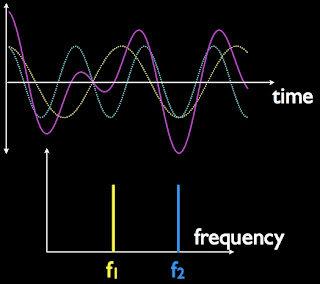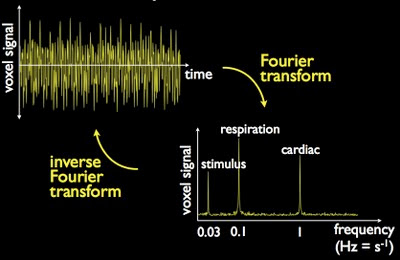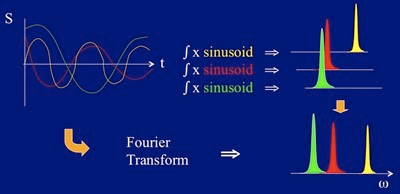Sure, you can try to fix it during data processing, but you're usually better off fixing the acquisition!

## Wednesday, June 15, 2011

### Physics for understanding fMRI artifacts: Part Five

An introduction to the Fourier transform - what it does and how it works

Moving along from bathroom design and complex numbers, it's time to look at one of the most fundamental mathematical relationships underpinning all of modern MRI: the Fourier transform (FT). Accordingly, I would strongly suggest that you make sure you fully understand everything in this post before continuing into future posts.

You may not believe it by looking at us, but take it from me that MR physicists do "mental FTs" every day as we compare pulse sequences, try to understand artifacts and so on. Many of us (me) are not mathematically inclined, either. But being able to switch your mind between domains, as they are called, is a really useful skill if you want to be good at artifact recognition. An MR physicist will see something in one domain and will immediately try to imagine how it would appear in the alternate domain. Thus, if an image contains an artifact - an intense stripe, say - the MR physicist tries to imagine what signal-acquisition domain feature is implied by it, then tries to track it down. Alternatively, when comparing two different pulse sequences - GRAPPA on versus GRAPPA off, perhaps - the MR physicist will project the implications of each into the image domain in order to comprehend the likely practical consequences. You don't have to remember the equations or even understand the maths itself, but it is really useful to grasp the concepts!

Fourier analysis: the art of decomposition

As you have seen in your introductory texts and in previous video lectures, MRI signals are actually time-varying voltages that are induced in receiver coils as a result of magnetization oscillating (precessing) about the polarizing magnetic field. The detection of time-varying signals has several implications for obtaining MR images, the very first of which is choosing a representation for the information content in the signals.

Consider the two waveforms on the left-hand side of the figure below, which have the same amplitude but differ in their frequency:Courtesy: Karla Miller, FMRIB, University of Oxford.

It is possible in principle - and in practice for simple examples such as these - to take a ruler and measure the amplitude and frequency and then draw a graph representing the time varying signals as a plot of amplitude (along y) against frequency (along x), as shown on the right-hand side of the above figure. Piece of cake, right?

But things get a lot more complicated, and our selection of a ruler as the analytic tool of choice gets a lot less useful, as soon as frequencies start to coalesce. Fancy trying to determine the amplitudes and frequencies of the constituents of this waveform?Courtesy: Karla Miller, FMRIB, University of Oxford.

It's not obvious to the untrained eye how many constituents the waveform possesses. Two? Three? Infinite...? Turns out there are only two:Courtesy: Karla Miller, FMRIB, University of Oxford.

And as soon as we have the constituent waveforms revealed to us as separate colors, we could again employ our trusty ruler to make some measurements and draw up a plot of amplitude versus frequency by hand. Still, this emphasizes the point that once multiple frequencies interfere, we have an analysis problem.

What about the heights of the lines in the frequency plot? It's obvious that if the amplitudes of the two individual waves are the same then the representations of them in the frequency plot should be the same also; only the frequencies differ. So, if one frequency has a lower amplitude than the other, we will see the following (compare the purple trace below to the one above):Courtesy: Karla Miller, FMRIB, University of Oxford.

Likewise, if the phases of the constituent waves change, the combined wave's shape changes even though the frequencies and amplitudes of the constituents are unchanged (see Note 1):The phase of the yellow signal differs between the left and right panels, causing the purple traces representing the combined signal to differ. Yet the frequencies in the two panels are actually the same, resulting in the same frequency plots in the bottom row. Courtesy: Karla Miller, FMRIB, University of Oxford.

There is one further property of the waves - decay - that we need to consider for completeness, but I don't want to get into finite signals in this post. Instead, for simplicity (and because it's all we need to understand how the FT works) I'm going to consider just frequency and amplitude for the time being. So, in the above examples, the waves are considered to have infinite duration; there is no attenuation of their amplitude with time, as is the case with real signals. We will consider decay in the next post. (See Note 2.)

Conjugate variables and domains

This alternate representation of waves by lines drawn on a graph of frequency is an example of what are called "domains." There is a special mathematical relationship between these two domains, and the FT allows us to switch between them with no loss (or gain) of information; they are equivalent.

Why should we want to do something that seems to have no net benefit (or loss)? Doesn't that make one or other of the domains redundant? It turns out that one domain is usually more convenient to work with than the other. For example, if you look at the real data example in the figure below you'd be hard pressed to recognize three distinct frequencies in the time domain! Clearly there is something repetitive in the time domain but a lot of it looks like noise! The constituents are clear in the frequency domain.

Time and frequency are an example of conjugate variables, sometimes also called a conjugate pair. They are variables that are directly related by an FT. A little bit of thought suggests why they might possess this property: frequency (1/sec)  is the reciprocal of time (sec), and vice versa, obviously. We will come back to another important pair of conjugate variables in a later post.

Which brings us to the magic tool - the Fourier transform - that allows us to swap between representations, from time domain to frequency domain, or vice versa. The information content in each domain is the same, so it is feasible to switch between domains ad infinitum:Courtesy: Karla Miller, FMRIB, University of Oxford.

How the Fourier transform works

Before getting into the mathematical representation of the FT, I want to illustrate its action and walk you through what it does. Let's take an "interferogram" (which is an archaic name NMR spectroscopists give to a time domain signal when we aren't calling it a free induction decay, or FID) and assume that we want to transform it into its frequency domain representation. The inteferogram and its three components are (badly) represented in the cartoon below (where I have made no attempt whatsoever to match the total amplitude on the left with the constituent amplitudes on the right - sorry about that):

Not only is the intensity on the left badly represented, I've also intentionally attenuated the waveforms after just a few cycles of each, so that it's easier to see the discrete frequencies. However, it is important to note that for the purposes of this illustration the waveforms should be considered to decay away to zero amplitude over a time period several times longer than I've shown. (As previously mentioned, we will consider the effect of "truncating" and decaying waveforms in the next post. For now, just assume that fully decaying waveforms are being manipulated.)

The wave in white on the left above doesn't look like either a sine or a cosine wave, but even though it's badly drawn I think it's reasonably clear that it is the sum of the three sinusoidal (or pure tone) waves on the right. Furthermore, if we assume that any waveform whatsoever, no matter how complicated, can ultimately be reduced to a set of constituents that are just sinusoids and cosinusoids (and their phase-shifted counterparts), then all we've got to do is identify the constituents! If you want an analogy, it's a little bit like trying to determine the elements (atoms) that make up a compound (molecules). By analogy, then, the Fourier transform is able to identify the subset of atoms in the target molecule because we know the molecule must consist of some of the elements to exist at all.

So now assume we have a magic tool which does the following set of operations:
1. If first defines a suitable range of frequencies that might reside in the interferogram. Let's say the range is 100 Hz, which is +/- 50 Hz centered at zero frequency. (See Note 3.)
2. It chops up the range of frequencies into equal digital chunks. Let's say that the chunks are each 1 Hz wide, so we have 100 possible frequencies to search for.
3. The interferogram is multiplied by a reference wave (a sinusoid, say) having a frequency of -50 Hz, and the resultant is then summed over time. If the interferogram has a component at -50 Hz then the result of the summation will be non-zero; if the interferogram doesn't have a component at -50 Hz then the magic tool returns zero.
4. The magic tool increments to the next frequency, -49 Hz, and repeats the multiplication by a reference waveform (a -49 Hz sinusoid) followed by summation over time. As before, the result is zero if there's no component at this reference frequency.
5. The process continues for each 1 Hz step through zero frequency and out to +50 Hz.
6. At the end of the 100 multiplications/summations the magic tool returns a plot of all the frequencies that were present in the interferogram. This is the frequency domain representation of the data.

Here is a cartoon of that operation in action, with each separate frequency color-coded:Illustration of how the FT determines the frequency content of a time domain signal.

There are two paths in this picture. The clockwise route illustrates the six steps outlined in the list above. The summation is done mathematically by integration, as denoted by the giant S-like symbols in white (middle, top row). The counter-clockwise route is labeled "Fourier Transform" and is the name of our magic tool; it produces the entire process listed above. Note also that in the cartoon I've included only three "successful" steps, i.e. where the interferogram did contain the reference frequency being sought. Imagine if you will a fourth reference sinusoid, in black, say, that is multiplied by the time domain signal and integrated. It would return no frequency domain peak because there's no black wave in the time domain. (There are one or two other explanatory points in Note 4, but feel free to read on if you wish.)

The mathematics of the Fourier transform

Here is the formula for the FT from the time domain to the frequency domain:

It looks a bit scary but it's actually remarkably simple. The interferogram is f(t) and the reference waves are given generally as exp(-i.w.t). (Why the negative sign? See Note 5.) The target waveform to be analyzed, f(t), is multiplied by a succession of reference waves over the limits -t to +t, as given above and below the integral symbol, and then the integral does the summing up to produce the frequency domain representation, F(w). (By convention we use upper-case symbols, F(w) to represent the Fourier transforms of target functions, f(t). Also see Note 6.)

Recall from Part Four of this series that it is possible to generate sinusoids or cosinusoids from the rotation of a vector, and that this relationship is encapsulated in Euler's formula. In essence, Euler's formula says that it is possible to represent any pure tone (either a sinusoid or a cosinusoid or a wave with some other phase) with a simple exponential term, exp(i.blah). (Note: strictly speaking there should be a factor of 2.pi radians in the exponent but I'll try to ignore it consistently; it doesn't help or hinder understanding, but it makes for less typing!)

Now review the exponent in the Fourier transform equation. If frequency (w) is in units of 1/sec and time is in units of sec then the units of our conjugate pair cancel to leave a term that is exp(-i.blah), where again blah is an angle and we're omitting the 2.pi radians. You should be able to see that the exponential term on the right-hand side of the FT equation thus represents all possible pure tone waves that could exist between the limits that are being imposed on the integration, -t to +t, because exp(-i.blah) = cos(blah) - i.sin(blah), according to Euler's formula. All frequencies that lie between -1/t and +1/t are "checked for" by the FT, as are all possible phases for these frequencies.

The appearance of the conjugate variables, time and frequency, in the exponential term of the FT equation, is probably the most important and useful feature of the mathematics for us, in practical terms. We will encounter a different pair of conjugate variables in a couple of posts' time, when we come to look at the spatial encoding that enables MR imaging. Next up, though, the practical consequences of a discrete (or digital) Fourier transform.

-------------------------

Notes:

1.  Phase can be represented in the frequency domain plots, too, but for simplicity the figures here show magnitude-only representations in the frequency domain. We may return to phase representation in the frequency domain in a later post, but since the vast majority of MRI and fMRI uses magnitude-only images (which are a representation of a 2-dimensional frequency domain) it's not something that most fMRIers ever have to worry about. That said, there can be some useful information in the phase of images, albeit with additional (excuse the pun....) complexity in processing and analysis. Something for an advanced post another day.

2.  When a time domain wave persists to infinity, with no decay, as shown (partially!) in the figures, the corresponding representation in the frequency domain is an infinitely sharp line. This line is known as a delta function in mathematical lexicon. Not that you really care right now, just know that when we get to real signals the representations in the frequency domain won't be infinitely narrow, they will be finite and there will be practical consequences.

3.  A negative frequency of -50 Hz, say, is simply 180 degrees phase shifted from the wave for +50 Hz. Thus, as we saw in the last post, a sine and cosine have a phase difference of 90 degrees, so it's possible to have "upside down" sines, say, which are zero amplitude at zero time and are at negative maximum a quarter cycle later in time; the same positive frequency would be at positive maximum a quarter cycle later.

4.  While the time domain representation is attenuated after just a few cycles of each wave, as already mentioned, the frequency domain representations are drawn accurately, wherein the width (at half height) of the lines drawn in the frequency domain reflect the rate of (full) decay of the signals in the time domain. As we'll see in detail in the next post, the faster the decay in the time domain, the broader the line in the frequency domain; it's a reciprocal relationship. So, since the fastest-decaying waveform of the three is the green frequency, its line in the frequency domain representation is broadest (at its half height). The slowest-decaying waveform of the three is the yellow frequency, hence its line is sharpest. The reciprocal relationship between decay rate in the time domain and linewidth in the frequency domain has profound implications for image resolution, which we'll see later.

5.  Convention gives the conversion of a time domain function into its frequency domain counterpart as the inverse Fourier transform, wherein the exponent is the complex conjugate of that in the (forward) Fourier transform. All of which is another way of saying that while this may annoy mathematicians and purists, as practical fMRIers we don't care. Forwards, backwards, whatever. The basic ideas are what count for us. If remembering that the FT is being done forwards or backwards was crucial then I probably couldn't have developed a reasonable career doing this stuff. And I can promise you, I can never remember which way I'm going! It's a flip-flop for goodness sake. Does it really matter if I'm flop-flipping sometimes? Nope. (An aside: I would also like to point out that the only time I ever think about spin and angular momentum is when I teach it to newbie fMRIers once a year. It's important stuff to have seen once, but it won't make any practical difference to your fMRI experiments! Trust me!)

6.  In the formula the limits of integration are between negative and positive infinity - all possible time. In practice, we limit the integral to a span of time during which we are actually measuring signal, i.e. the sampling period. Furthermore, the Fourier transform as given in the formula is continuous, whereas in practice we use a digitized signal, and we therefore use what's called a discrete Fourier transform. These twin issues - a finite sampling period and digitization - lead to certain experimental consequences that we will see in the next post. But for the sake of comprehending the properties of the FT at this stage, the continuous FT is perfectly fine, perhaps slightly easier.